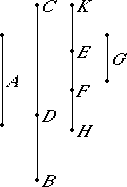# Proposition 113

The square on a rational straight line, if applied to an apotome, produces as breadth the binomial straight line the terms of which are commensurable with the terms of the apotome and in the same ratio; and further the binomial so arising has the same order as the apotome.

Let A be a rational straight line and BD an apotome, and let the rectangle BD by KH equal the square on A, so that the square on the rational straight line A when applied to the apotome BD produces KH as breadth.

I say that KH is a binomial straight line the terms of which are commensurable with the terms of BD and in the same ratio, and further, KH has the same order as BD.X.73

Let DC be the annex to BD. Then BC and CD are rational straight lines commensurable in square only. Let the rectangle BC by G also equal the square on A.

X.20

But the square on A is rational, therefore the rectangle BC by G is also rational. And it has been applied to the rational straight line BC, therefore G is rational and commensurable in length with BC.

Since now the rectangle BC by G equals the rectangle BD by KH, therefore, CB is to BD as KH is to G. But BC is greater than BD, therefore KH is also greater than G.

But BC is greater than BD, therefore KH is also greater than G.

Make KE equal to G. Then KE is commensurable in length with BC.

V.19,Cor.

Since CB is to BD as HK is to KE, therefore, in conversion, BC is to CD as KH is to HE.

V.19

Let it be contrived that KH is to HE as HF is to FE. Then the remainder KF is to FH as KH is to HE, that is BC is to CD.

V.11

But BC and CD are commensurable in square only, therefore KF and FH are also commensurable in square only.

Since KH is to HE as KF is to FH, while KH is to HE as HF is to FE, therefore KF is to FH as HF is to FE, so that also the first is to the third as the square on the first to the square on the second. Therefore KF is to FE as the square on KF is to the square on FH.

But the square on KF is commensurable with the square on FH, for KF and FH are commensurable in square, therefore KF is also commensurable in length with FE, so that KF is also commensurable in length with KE.

X.12

But KE is rational and commensurable in length with BC, therefore KF is also rational and commensurable in length with BC.

V.16

Since BC is to CD as KF is to FH, alternately, BC is to KF as DC is to FH.

X.11

But BC is commensurable with KF, therefore FH is also commensurable in length with CD.

But BC and CD are rational straight lines commensurable in square only, therefore KF and FH are also rational straight lines commensurable in square only. Therefore KH is binomial.

X.14

If now the square on BC is greater than the square on CD by the square on a straight line commensurable with BC, then the square on KF is also greater than the square on FH by the square on a straight line commensurable with KF.

And, if BC is commensurable in length with the rational straight line set out, then KF is also; if CD is commensurable in length with the rational straight line set out, then FH is also; but, if neither of the straight lines BC nor CD, then neither of the straight lines KF nor FH.

X.14

But, if the square on BC is greater than the square on CD by the square on a straight line incommensurable with BC, then the square on KF is also greater than the square on FH by the square on a straight line incommensurable with KF.

And, if BC is commensurable with the rational straight line set out, then KF is also; if CD is so commensurable, then FH is also; but, if neither of the straight lines BC nor CD, then neither of the straight lines KF nor FH.

Therefore KH is a binomial straight line, the terms of which KF and FH are commensurable with the terms BC and CD of the apotome and in the same ratio, and further, KH has the same order as BD.

Therefore, the square on a rational straight line, if applied to an apotome, produces as breadth the binomial straight line the terms of which are commensurable with the terms of the apotome and in the same ratio; and further the binomial so arising has the same order as the apotome.

Q.E.D.

## Guide

This proposition is not used in the rest of the Elements.